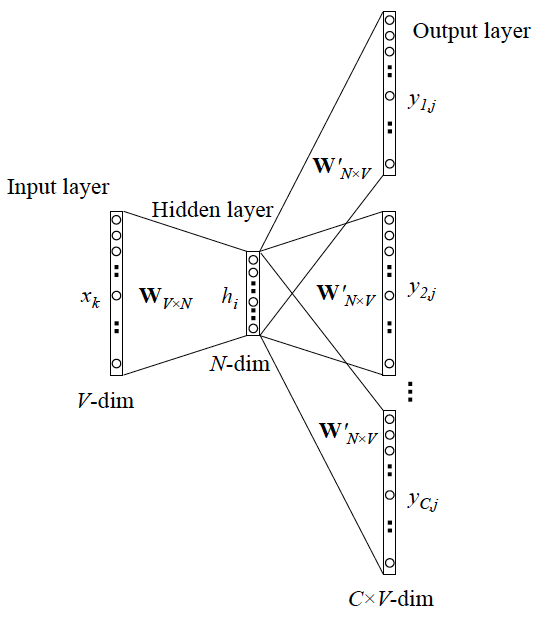# Natural Language Processing Lecture05

## Word2Vec

• 每个单词的意思是⼀个向量，v1,v2,v3,..vN
• 前⽂中所述的 function 也是⼀个神经⽹络线性变化，F
• 对于⽂章中的每个单词 C，使⽤ C 的上下⽂单词，v_i,v_i+1,..v_k 送⼊ F 中，使得 $F(v_i,v_{i+1},..v_k)$ 产出⼀个概率分布，该概率分布中，使得 C 的位置值的概率值最⼤
• 使⽤ backpropagation 不断优化 v_1,v_2,..v_n 的值，以及 F 的值
• 不断更新各个向量以及F的值，使得预测概率分布的准确性越来越⾼
• 则，我们获得了⼀个函数 F(context_words)=具体某个单词
• 对于某个单词 v-k，假设它有两组上下⽂：
• $v_i,v_j,v-k,v_m,v_n$
• $v_i,v_j,v-k,v_p,v_n$
• $F(v_1,v_j,v_m,v_n)=F(v_i,v_j,v_p,v_n)$
• 则，$v_m=v_p$
• V-m 和 v-p 的上下⽂类似，经过不断的迭代更新，其向量也⼀样了，⽽上下⽂类似，说明其语义类似
• 所以，v-m 和 v-p 的语义⼀样，⽽向量表⽰也⼀样了
• Two model variants
• Skip-Gram (SG): Predict context ("outside") words (position independent) given center word
• 用一个词语作为输入，来预测它周围的上下文
• Continuous Bag of Words (CBOW): Predict center word from (bag of) context words
• 拿一个词语的上下文作为输入，来预测这个词语本身## Skip Gram ModelFor each position c=1,…,T, predicate context words within a window of fixed size m, given center word $w_c$, given parameters θ

$Likehood(\theta)=\prod_{c=1}^T\prod_{-m\le j \le m,j\neq0}Pr(w_{c+j}|w_c;\theta)$

$Loss(\theta)=-\frac1TLogLikehood(\theta)=-\frac1T\sum_{t=1}^T\sum_{-m\le j \le m,j\neq0}log(Pr(w_{c+j})|w_c;\theta)$

$Pr(w_{c+j})=\frac{\vec{e}^T_{c+j}\cdot\vec{c}}{\sum_{w\in W \ e^{\vec{v_w^T \cdot \vec{v_C}}} }}$

• Loss函数趋向于 0 <=> 拟合的函数 + 向量，根据上下文预测单词的能力越强

## Softmax

Softmax 函数可以把任意给定的向量 X 变成概率分布：

• Max：把 X 中最大的 $x_i$ 值变得更大
• Soft：依然对较小的值会给到权重

### Hierarchical Softmax### Negative Sampling

$E=-log(\sigma(u_0^Tv_c))+\sum_{j-P(W)}[log\sigma (-u^T_jv_c)]$

## Other WordEmbedding

### Glove (Global Vector)### CoVe (Context Vector)### EMLO (Embedding of Language Model)## Sentence Embedding

Weighted Bag-of-words + remove some special direction

• Step1: 构建平滑的加权词向量
• Step2: 计算 PCA，减去最明显的第一个维度

https://openreview.net/pdf?id=SyK00v5xx

# NLP